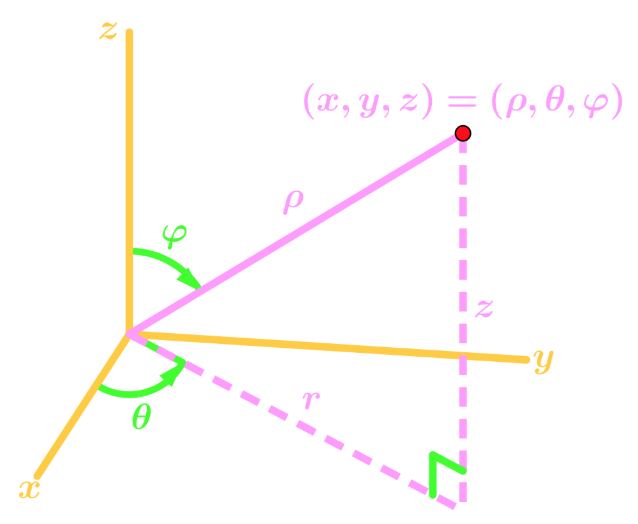# Triple integrals in spherical coordinates

### Triple integrals in spherical coordinates

#### Lessons

Notes:

Spherical Coordinates

There are times where instead of Cartesian coordinates, we use spherical coordinates for triple integrals. For spherical coordinates, instead of $x,y,z$'s, we have $\rho, \theta, \varphi$'s. In other words,

$(x,y,z) \to (\rho, \theta, \varphi )$Equations to Convert From Cartesian to Spherical

From the graph, we can obtain the following equations which will be useful for converting spherical to cartesian, or vice versa:

$r = \rho \sin \varphi$
$z = \rho \cos \varphi$
$z^2 + r^2 = \rho^2$

Using the equations from past sections, we can also obtain more equations:

$x = \rho \sin \varphi \cos \theta$
$y = \rho \sin \varphi \sin \theta$
$\rho^2 = x^2 + y^2 + z^2$

Triple Integrals in Spherical Coordinates

Suppose we want to convert a triple integral of $f(x,y,z)$ in Cartesian Coordinates to spherical Coordinates on region $E$. Let region $E$ be:

$a \leq \rho \leq b$
$\alpha \leq \theta \leq \beta$
$\delta \leq \varphi \leq \gamma$

Then the conversion would be:

$\int \int \int_E f(x,y,z)dV = \int_\delta^\gamma \int_\alpha^\beta \int_a^b f(\rho \sin \varphi \cos \theta, \rho \sin \varphi \sin \theta, \rho \cos \varphi)\rho^2 \sin \varphi d p d\theta d\varphi$

• Introduction
Triple Integrals in Spherical Coordinates Overview:
a)
Spherical Coordinates
• $(x,y,z) \to (\rho, \theta, \varphi )$
• Graph of the coordinates in 3D

b)
Equations to Convert from Cartesian to Spherical
• Pythagoras
• Trig Ratios

c)
Triple Integrals in Spherical Coordinates
• Region $E$
• Add an extra $\rho^2 \sin \varphi$
• An Example

• 1.
Convert the following integral into spherical coordinates

$\large \int_{-\frac{3}{2}}^{\frac{3}{2}}\int_{-\sqrt{\frac{9}{4}} - x^{2}}^{\sqrt{\frac{9}{4} - x^{2}}}\int_{\sqrt{3x^{2} + 3y^{2}}}^{\sqrt{9 - x^{2} - y^{2}}} \, x^{3} + xy^{2} + xz^{2} \,dz \,dy \,dx$

• 2.
Convert the following integral into spherical coordinates

$\large \int_{ -\sqrt{2} }^{0} \int_{-\sqrt{2 - x^{2}}}^ {0} \int_{\sqrt{x^{2} + y^{2}}}^ {\sqrt{4 - x^{2} - y^{2}}} \, x + y + dz \, dy \,dx$

• 3.
Evaluating Triple Integrals in Spherical Coordinates
Evaluate $\int\int\int_{E}2x^{2} + 2y^{2} \, dV \,$ where $E$ is the region portion of $x^{2} + y^{2} +z^{2} = 1\,$ with $z \geq 0$.

• 4.
Evaluating Triple Integrals in Spherical Coordinates
Evaluate $\int\int\int_{E}3 \, dV \,$ where $E$ is the region bounded by $x^{2} + y^{2} +z^{2} = 4$ and $z = -\sqrt{x^{2} + y^{2}}$.